# FEA Analysis in SOLIDWORKS Sample

Instructions:

Perform a simple FEA simulation using the SOLIDWORKS software. Design the geometry of a solid body and apply the material, appropriate boundary conditions, and external loads in order to calculate the maximal strain in the body. Compare the result with the analytical solution.

Solution: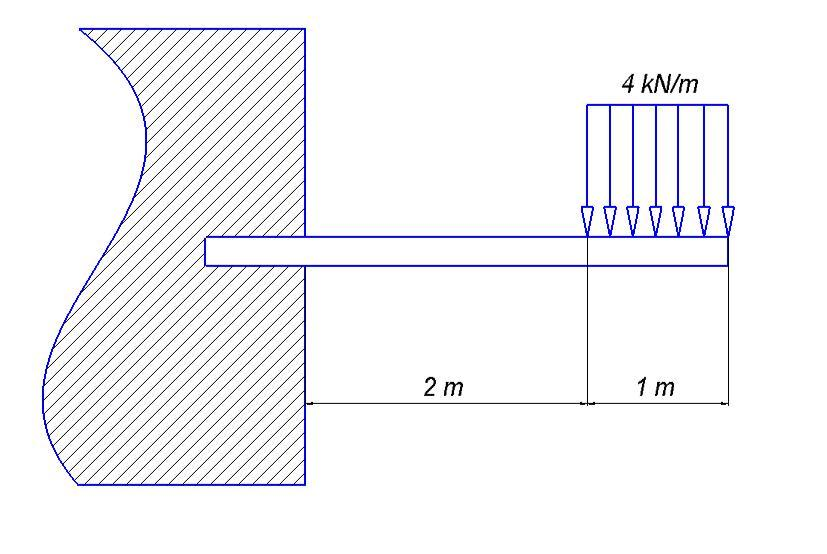The cross-section is assumed to be a simple rectangle with a 150 mm height and 50 mm width.

Then, the first step will be to create the cross-sectional area in the arbitrarily selected sketch: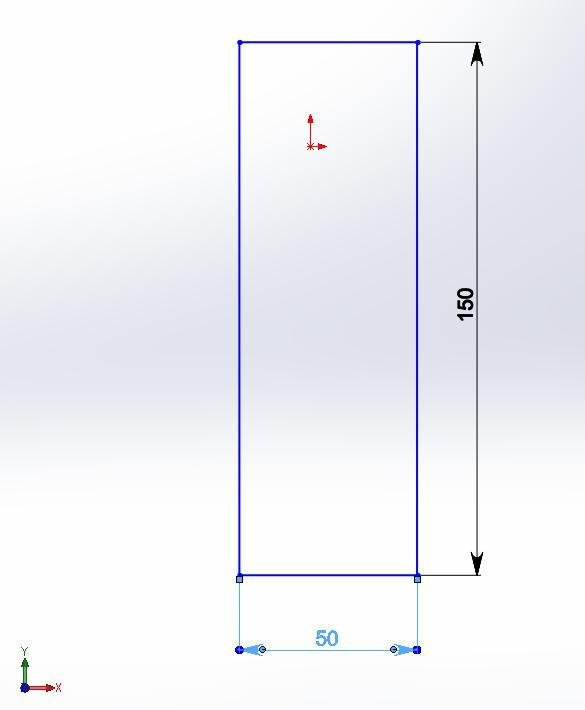The next step will be an extrusion of 3 m length of a solid beam: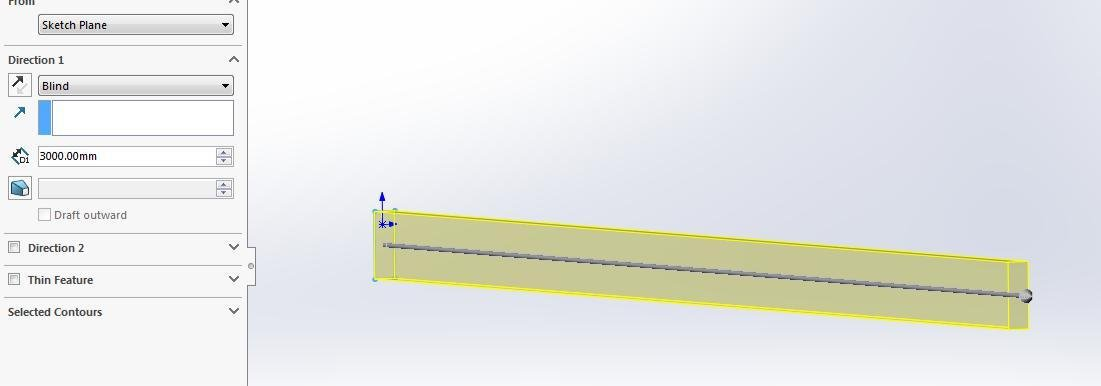After that, a static analysis should be run by selecting the appropriate case study in the SOLIDWORKS window: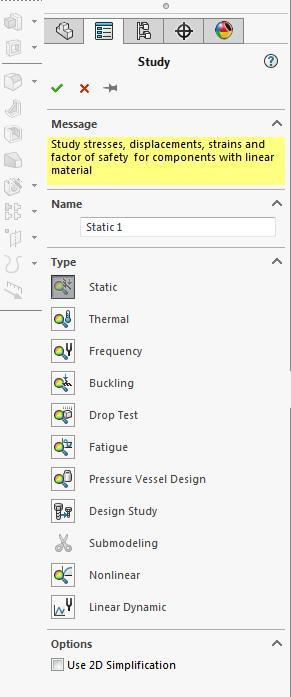It should be noted that the 1 m (or 1000 mm) of free length needs to be separated from the whole surface for the load application.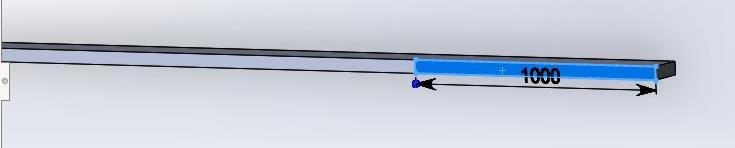As for the boundary conditions, the left side of the beam should be fixed and the right span of 1 m should be loaded by the distributed load of 4 kN/m.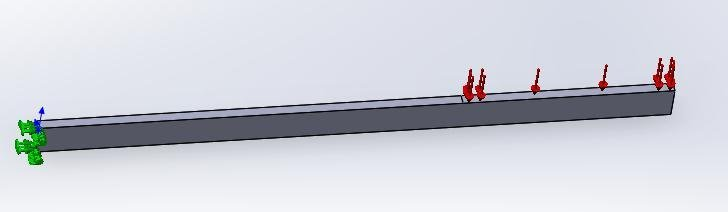Let’s assume the material for this body to be 6063-O Aluminum Alloy.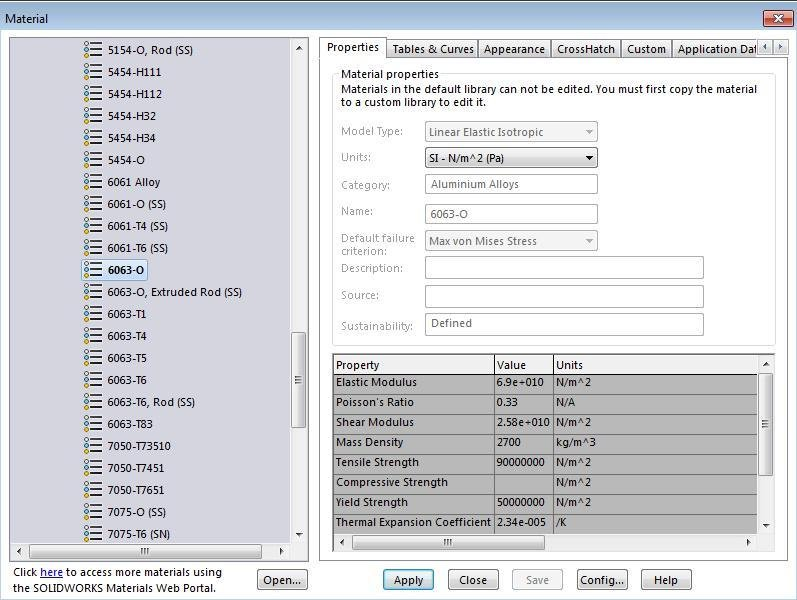A standard mesh will be built there. Since the geometry is very simple, there is no need for a small mesh size.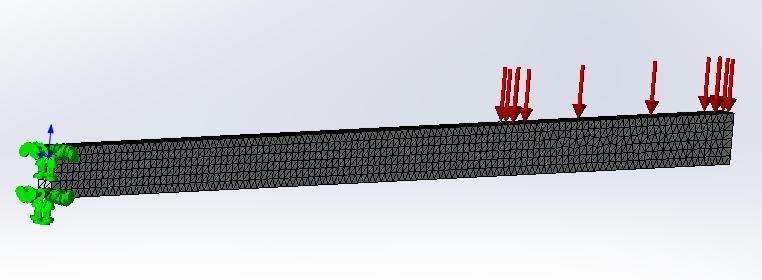After running the simulation analysis, the following result will be shown: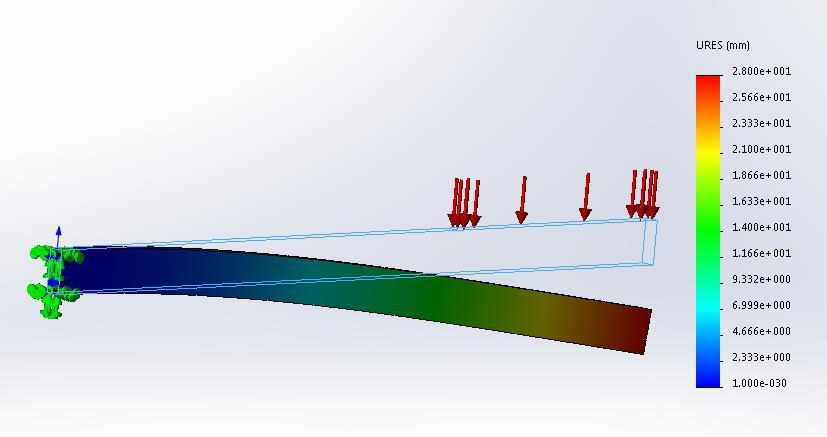As can be seen, the maximal displacement is: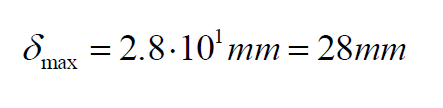Let’s check the analytical solution. For this purpose, use the Area-Moment method: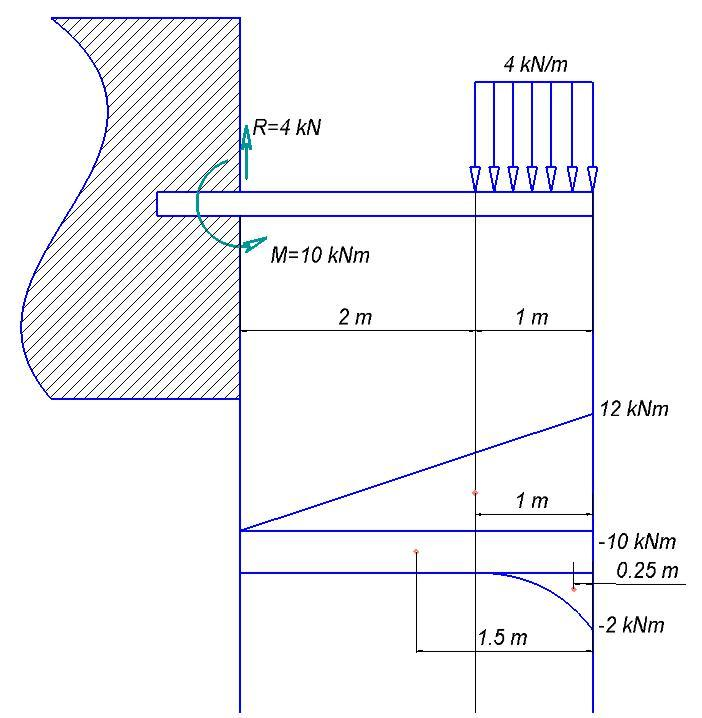The deflection of the end can be calculated as: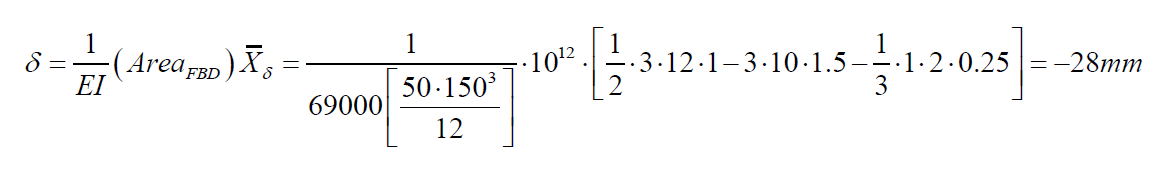As can be seen, the calculated value equals 28 mm. Thus, the FEA provided the exact solution compared to the analytical analysis. However, a real-life experiment could provide a different value, since the real-life beam has non-homogenous properties and non-linear behavior.

Most students will find this FEA analysis in SOLIDWORKS helpful. It was completed by one of our experts knowledgeable in the topic. The analysis is helpful if this topic is not quite clear to you and you don’t know what to do with your assignment. However, using a solidworks FEA analysis tutorial is not the only way to succeed.

One more way is to get help from our experts. If you want to be sure that you will get a good grade, place an order on our site. When our experts are dealing with your assignment, you can spend your time as you like. Many people have already used our service and remain satisfied with it. You can become one of them by requesting help at Assignment.EssayShark.com.